# Class 10 Science: CBSE Sample Question Paper- Term II (2021-22) - 4 Notes | Study CBSE Sample Papers For Class 10 - Class 10

## Class 10: Class 10 Science: CBSE Sample Question Paper- Term II (2021-22) - 4 Notes | Study CBSE Sample Papers For Class 10 - Class 10

The document Class 10 Science: CBSE Sample Question Paper- Term II (2021-22) - 4 Notes | Study CBSE Sample Papers For Class 10 - Class 10 is a part of the Class 10 Course CBSE Sample Papers For Class 10.
All you need of Class 10 at this link: Class 10

Class-X

Time: 120 Minutes
M.M: 40

General Instructions:

1. All questions are compulsory.
2. The question paper has three sections and 15 questions.
3. Section–A has 7 questions of 2 marks each; Section–B has 6 questions of 3 marks each; and Section–C has 2 case based questions of 4 marks each.
4. Internal choices have been provided in some questions. A student has to attempt only one of the alternatives in such questions.
Section - A

Q.1. Carbon is a Group 14 element in the Periodic Table. It is known to form compounds with many elements. Write an example of a compound formed with:
(a) Chlorine, which is a group 17 element of a Periodic Table.
(b) Oxygen, which is a group 16 element of a Periodic Table.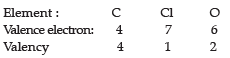(a) Thus, carbon can share its four electrons with four chlorine atoms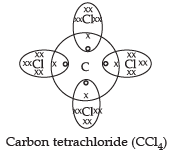(b) Carbon dioxide (CO2)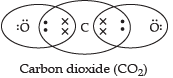Thus, carbon can share its four electrons with two oxygen atoms.

Q.2. Choose from the following:
20Ca, 3Li, 11Na, 10Ne
(a) An element having two shells completely filled with electrons.
(b) Two elements belonging to the same group of the periodic table.

(a) 10Ne has electronic configuration 2, 8. It’s both shells (shell k, L) are completely filled.
(b) 3Li and 11Na belong to same group of the periodic table, i.e. 1st group.

Q.3. (a) What is the pathway of sperm during ejaculation?
(b) Name the organs producing sperms and ova respectively in humans.

(a) Sperms come out from testis into the vas deferens and pass through the urethra before ejaculation. The secretions of seminal vesicle and prostate glands provide nutrition to the sperms and also facilitate their transport.
(b) Testis: Sperms, Ovary: Ova.

Q.4. Shweta conducted a study on a tobacco plant. She found that in tobacco plant, the male gametes have twenty four chromosomes. Based on this information, find out the number of chromosomes in:
(a) Female gamete
(b) Zygote.

(a) Number of chromosomes in female gamete is 24.
(b) Number of chromosomes in zygote is 48.

Q.5. In a monohybrid cross between tall pea plants (TT) and short pea plants (tt), a scientist obtained only tall pea plants (Tt) in the F1 generation. However, on selfing the F1 generation pea plants, he obtained both tall and short plants in F2 generation. On the basis of above observations with other angiosperms, can the scientist arrive at a law? If yes, explain the law. If not, give justification for your answer.
OR
‘A trait may be inherited, but may not be expressed.’ Justify this statement with the help of a suitable example.

Yes, the scientist may arrive at the law of dominance according to which the trait that is expressed in the F1 generation is the dominant trait, although both the dominant and recessive traits are present in the F1 generation. In the F2 generation, the recessive traits are also expressed along with the dominant traits.
OR
When pure tall pea plants are crossed with pure dwarf pea plants, only tall pea plants are obtained in F1 generation.
On selfing tall plants of F1, both tall and dwarf plants are obtained in F2 generation in the ratio 3:1.
Reappearance of the dwarf character, a recessive trait in F2 generation shows that the dwarf trait/character was present in individuals of F1 generation but it did not express (due to the presence of tallness, a dominant trait/character).

Q.6. Varun divided a magnet into three parts A, B, and C.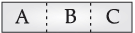(a) Name the parts where the strength of the magnetic field is:
(i) maximum
(ii) minimum
(b) The density of magnetic field lines differ at these parts. Explain.
OR
Priyanka arranged two magnets side by side as shown below.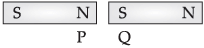(a) Draw magnetic field line between poles P and Q.
(b) What does the degree of closeness of magnetic field lines near the poles signify?

(a) (i) Maximum strength – At A and C
(ii) Minimum strength – At B
(b) At poles A and C, magnetic field lines are crowded that diverges from poles, so magnetic density is high at the poles.
While at B, they are parallel to the centre and hence magnetic effect is minimum.
OR
(a)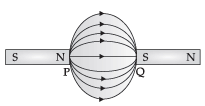(b) The degree of closeness of magnetic field lines near the poles signify that the field is stronger there.

Q.7. Shilpa studied the flow of energy at different trophic levels. Based on her studies she draw a flow chart. What does this flowchart depict? Why a food chain cannot have more than four trophic levels?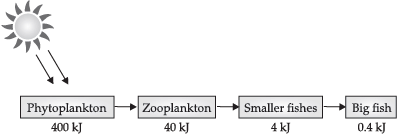OR
In the given figure, the various trophic levels are shown in a pyramid. What does "X" and "Z" represent?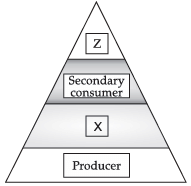It is a food chain that is depicting 10% law of energy.
The loss of energy at each step is so great that very little usable energy remains after four trophic levels.
OR
X: Primary consumer.
Z: Tertiary consumer.

Section - B

Q.8. Three elements are represented in the given periodic table: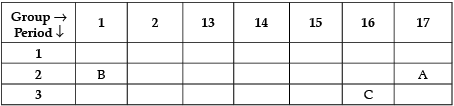By giving reasons, explain the following questions:
(a) Element A is non-metal.
(b) Atom of element C has a larger size than atom of element A.
(c) Element B has a valency of 1.

(a) It is because it has 7 valence electrons.
It can gain one electron to form negative ion. So, it is a non-metal.
(b) ’C’ has more number of shells than 'A'. So, it is larger in size.
(c) ’B’ has one valence electron. It can lose one electron to become stable. So, its valency is equal to 1.

Q.9. (a) Elements that form ionic compounds attain noble gas electronic configuration by either gaining or losing electrons from their valence shells. Explain by giving reason why carbon cannot attain such a configuration in this manner to form its compounds.
(b) Name the type of bonds formed in ionic compounds and in the compounds formed by carbon.
OR
(a) What are isomers? Draw the structures of two isomers of butane, C4H10
(b) Why can’t we have isomers of first three members of alkane series?

(a) Carbon has 4 electrons in its outermost shell. It cannot lose 4 electrons to form C4+ as very high energy is required to remove 4 electrons from its valence shell.
It cannot gain 4 electrons to form C4– as it is difficult for 6 protons to hold on to 10 electrons.
(b) In ionic compounds, ionic bonds are formed; while in carbon compounds, covalent bonds are formed.
OR
(a)
Those compounds, which have same molecular formula but different structural formulae, are called isomers.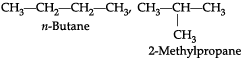(b) Isomerism is not possible in the first 3 alkanes primarily because branching is not possible from the first or the last carbon atom of the structure.

Q.10. Shivani tried to cross a homozygous purple flower variety of pea plant (PP) with white flower variety of pea plant (pp). Mention the phenotype and genotype of the F1 generation of off springs. If the off springs of the F1 generation are self bred. What will be the phenotypic and genotypic ratios of the F2 generation ? State Mendel's law of dominance.

In Fgeneration:

• Phenotypes: All bear purple flower
• Genotype: All are heterozygous dominant for purple flower i.e., Pp

In Fgeneration:

• Phenotypic ratio: 3:1
• Genotypic ratio: 1:2:1

Law of Dominance: Out of a pair of contrasting characters, only one is able to express while the other remains suppressed.

Q.11. (a) What is Electric power? Express it in terms of potential difference V and resistance R.
(b) An electric iron of 1 kW is operated at 220 V. Find, which of the following fuses that respectively rated at 1 A, 3 A and 5 A can be used in it?

(a) Electric power: It is the rate of doing work by an energy source or the rate at which the electrical energy is dissipated or consumed per unit time in the electric circuit.
So, Power, P = Work done (W)/Time (t)
= Electrical energy dissipated/Time (t)
= VIt/t
= VI = V2/R [∵ I = (V/R)]
(b) Given: P = 1 kW = 1000 W, V = 220 V
Current drawn, I = P/V = 1000/220 = 50/11
= 4.54 A
To run electric iron of 1 kW, rated fuse of 5 A should be used.

Q.12. The figure below shows three cylindrical copper conductors along with their face areas and lengths. Compare the resistance and the resistivity of the three conductors. Justify your answer.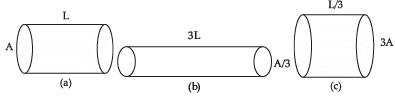OR
Consider the following circuit: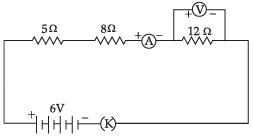Calculate the readings of the ammeter and the voltmeter when key 'K' is closed. Give reason to justify your answer.

Ra = ρ(L/A)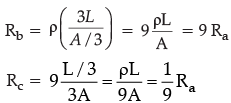Hence Rb > Ra > Rc
ρa = ρb = ρc because all the three conductors are of same material.
For the figure a,
Length of the cylinder = L
Cross sectional area of the cylinder = A
Now we will simply put these values in the formula to find out resistance.
So, we get, Ra = ρ(L/A)
For the figure (b),
Length of the cylinder = 3L
Cross sectional area of the cylinder = A/3
Now we will simply put these values in the formula to find out resistance.
So, we get Rb =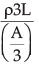We can rewrite this equation as
Rb = ρ(9L/A)
For figure (c),
Length of the cylinder = L/3
Cross sectional area of the cylinder = 3A
Now, we will simply put these values in the formula to find out resistance.
So, we get R=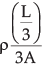We can rewrite this equation as
Rc = 1/9 (ρ(L/A))
Comparing Ra, Rb and Rc, we can observe that Rb = 9Ra and Rc = (1/9)Ra
Therefore, we can arrange the resistances in the following order Rb > Ra > Rc
Since all conductors are made by copper (same material), so their resistivity will remain same i.e., ρ= ρb = ρc
OR
R = R1 + R2 + R3
R = 5 Ω + 8 Ω + 12 Ω
= 25 Ω
Given, V = 6V
By Ohm's law, V = IR
∴ I = V/R = 6V/25Ω = 0.24 A
Hence, the ammeter reading will be = 0.24 A
∴  Voltage across 12 Ω resistor = IR
= 0.24 × 12 = 2.88
Hence, the voltmeter reading will be 2.88 V.

Q.13. Management of waste is very important in today's society. The generation of waste is getting doubled day by day, as the population is increasing continuously. Moreover, the increase in waste is affecting the lives of many people.
(a) Differentiate between biodegradable and non-biodegradable substances with the help of one example each.
(b) List two changes in habit that people must adopt to dispose non-biodegradable waste, for saving the environment.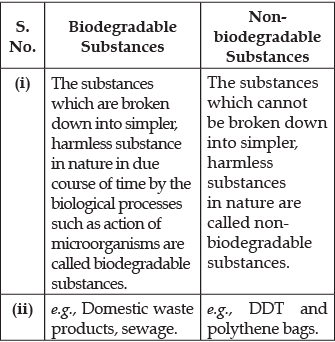(b) Two methods of disposal of non-boiodegradable waste are:
(i) Recycling: The wastes are treated and same value materials are extracted for reuse.
(ii) Incineration: Medical and toxic waste are burnt at high temperature in  Incinerators. Incinerators transform the waste into ashes.

Section - C

This section has 02 case-based questions (14 and 15). Each case is followed by 03 sub-questions (a, b and c). Parts a and b are compulsory. However, an internal choice has been provided in part c.

Q.14. Seema crossed pure breed of pea plants having round-yellow seeds with wrinkled green seeds and found that only A – B type of seeds were produced in the F1 generation. When in F1 generation, pea plants having A – B type of seeds were crossbred by self pollination, then in addition to the original round yellow and wrinkled green seeds, two new varieties A – D and C – B types of seeds were also obtained.
(a) What are A – B type of seeds?
(b) State whether A and B are dominant traits or recessive traits.
(c) What are A – D and C – B type of seeds?
OR
Which one will be produced in minimum and maximum number in the F2 generation?
(a) A – B
(b) C – D

(a) A – B type of seeds – Round in shape and yellow in colour.
(b) A – B type is dominant traits.
(c) A – D = Round-green
C – B = Wrinkled- yellow
OR
C – D in minimum number
A – B in maximum number

Q.15. Asif studied about electromagnetic current induced by changing magnetic field in the class. In the physics laboratory, he experimented the concept. He took two coils of insulated copper wire and wound it over a non conducting cylinder as shown. Coil P had larger number of turns than coil S.. He observed some deflections in the galvanometer and read it.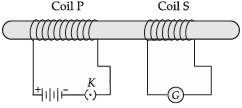(a) What did he observed when,
(i) key K was closed;
(ii) Key K was opened.
(b) Give reason for his observations.
(c) Define the phenomenon involved in the activity.
OR
What will happen if the number of turns in the coil S in increased?

(a) (i) A momentary deflection was shown by the galvanometer.
(ii) A  momentary deflection was shown by the galvanometer but in the opposite direction.
(b) When key was closed or opened, the current in the coil P changed, therefore the magnetic field linked with coil S will  change and hence a current was induced in it.
(c) Electromagnetic induction: The process by which a changing magnetic field in a conductor induces current in another conductor.
OR
If the number of turns in coil S is increase, the induced current will be more and the deflection of the galvanometer will also be more.

The document Class 10 Science: CBSE Sample Question Paper- Term II (2021-22) - 4 Notes | Study CBSE Sample Papers For Class 10 - Class 10 is a part of the Class 10 Course CBSE Sample Papers For Class 10.
All you need of Class 10 at this link: Class 10Use Code STAYHOME200 and get INR 200 additional OFF

## CBSE Sample Papers For Class 10

174 docs|7 tests

Track your progress, build streaks, highlight & save important lessons and more!

,

,

,

,

,

,

,

,

,

,

,

,

,

,

,

,

,

,

,

,

,

;